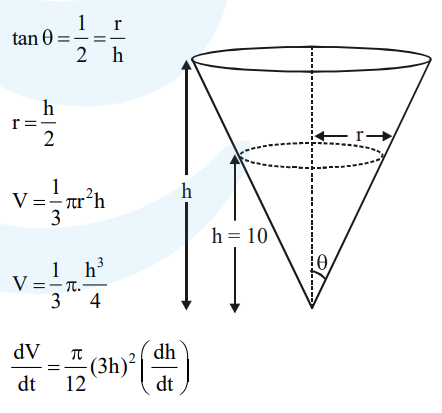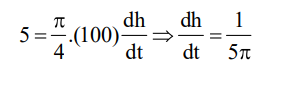# A water tank has the shape of an inverted right circular cone, whose semi-vertical angle is`
Question:

A water tank has the shape of an inverted right circular cone, whose semi-vertical angle is

$\tan ^{-1}\left(\frac{1}{2}\right) .$ Water is poured into it at a constant

rage of 5 cubic meter per minute. The the rate (in $\mathrm{m} / \mathrm{min}$.), at which the level of water is rising at the instant when the depth of water in the tank is $10 \mathrm{~m}$; is :-

1. $2 / \pi$

2. $1 / 5 \pi$

3. $1 / 10 \pi$

4. $1 / 15 \pi$

Correct Option: , 2

Solution: